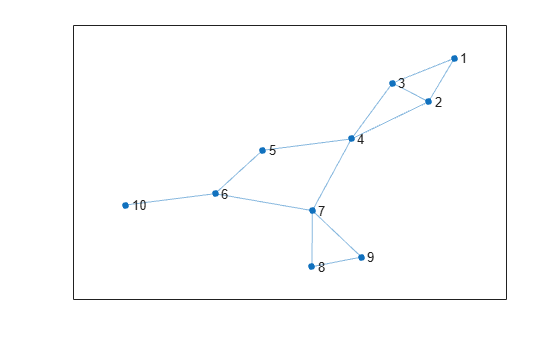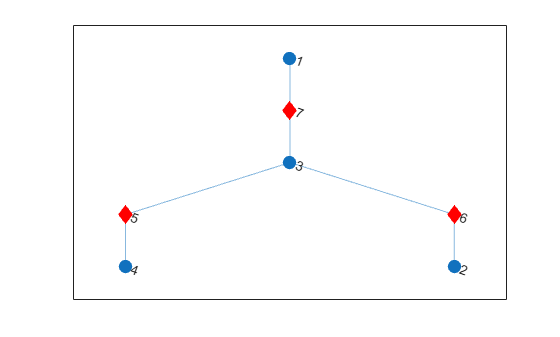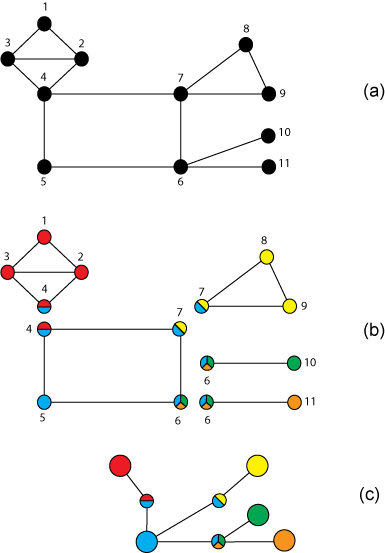# bctree

Block-cut tree graph

## Syntax

``tree = bctree(G)``
``````[tree,ind] = bctree(G)``````

## Description

example

````tree = bctree(G)` returns the block-cut tree of graph `G`, such that each node in `tree` represents either a biconnected component or cut vertex of `G`. A node representing a cut vertex is connected to all nodes representing biconnected components that contain that cut vertex.```

example

``````[tree,ind] = bctree(G)``` also returns a vector of numeric node indices mapping the nodes of `G` into the nodes of `tree`.```

## Examples

collapse all

Compute the block-cut tree of a graph, view the resulting node properties, and then highlight the cut vertices in the graph plot.

Create and plot a graph.

```s = [1 1 2 2 3 4 4 5 6 6 7 7 8]; t = [2 3 3 4 4 5 7 6 7 10 8 9 9]; G = graph(s,t); p = plot(G);```Compute the block-cut tree of the graph and view the node properties.

```tree = bctree(G); tree.Nodes```
```ans=7×3 table IsComponent ComponentIndex CutVertexIndex ___________ ______________ ______________ true 1 0 true 2 0 true 3 0 true 4 0 false 0 4 false 0 6 false 0 7 ```

Plot the block-cut tree using red diamond markers for the nodes that represent cut vertices. The circular nodes represent the biconnected components in the original graph.

```p2 = plot(tree,'MarkerSize',9); highlight(p2,5:7,'Marker','d','NodeColor','r')```Create and plot a graph.

```s = [1 1 2 2 3 4 4 5 6 6 7 7 8]; t = [2 3 3 4 4 5 7 6 7 10 8 9 9]; G = graph(s,t); p = plot(G);```Compute the block-cut tree `tr` of the graph, and specify a second output `ix` to return the node indices.

`[tr,ix] = bctree(G)`
```tr = graph with properties: Edges: [6x1 table] Nodes: [7x3 table] ```
```ix = 1×10 4 4 4 5 3 6 7 1 1 2 ```

Each index `ix(j)` indicates the node in the block-cut tree that represents node `j` in the input graph. For example, node 4 in `tr` represents a component in `G` that contains nodes 1, 2, and 3, so the first three entries in `ix` are all 4.

## Input Arguments

collapse all

Input graph, specified as a `graph` object. Use `graph` to create an undirected graph object.

Example: `G = graph(1,2)`

## Output Arguments

collapse all

Block-cut tree graph, returned as a `graph` object. `tree` contains a node for each cut vertex in `G` and a node for each biconnected component in `G`. The nodes table `tree.Nodes` contains additional node attributes to describe what each node represents:

• `tree.Nodes.IsComponent(i)` — Value equal to logical `1` (`true`) if node `i` represents a biconnected component. Otherwise, the value is equal to and logical `0` (`false`).

• `tree.Nodes.ComponentIndex(i)` — Index indicating the component represented by node `i`. The value is zero if node `i` represents a cut vertex.

• `tree.Nodes.CutVertexIndex(i)` — Index indicating the cut vertex represented by node `i`. The value is zero if node `i` represents a biconnected component.

Node indices, returned as a numeric vector. `ind(i)` is the node in the output graph `tree` that represents node `i` in the input graph `G`:

• If node `i` is a cut vertex in graph `G`, then `ind(i)` is the associated node in `tree`.

• If node `i` is not a cut vertex, but belongs to one of the biconnected components in graph `G`, then `ind(i)` is the node in `tree` representing that biconnected component.

• If node `i` is an isolated node in graph `G`, then `ind(i)` is zero.

collapse all

### Biconnected Components

A biconnected component of a graph is a maximally biconnected subgraph. A graph is biconnected if it does not contain any cut vertices.

Decomposing a graph into its biconnected components helps to measure how well-connected the graph is. You can decompose any connected graph into a tree of biconnected components, called the block-cut tree. The blocks in the tree are attached at shared vertices, which are the cut vertices.

The illustration depicts:

• (a) An undirected graph with 11 nodes.

• (b) Five biconnected components of the graph, with the cut vertices of the original graph colored for each component to which they belong.

• (c) Block-cut tree of the graph, which contains a node for each biconnected component (as large circles) and a node for each cut vertex (as smaller multicolored circles). In the block-cut tree, an edge connects each cut vertex to each component to which it belongs.### Cut Vertices

Also known as articulation points, cut vertices are graph nodes whose removal increases the number of connected components. In the previous illustration, the cut vertices are those nodes with more than one color: nodes 4, 6, and 7.